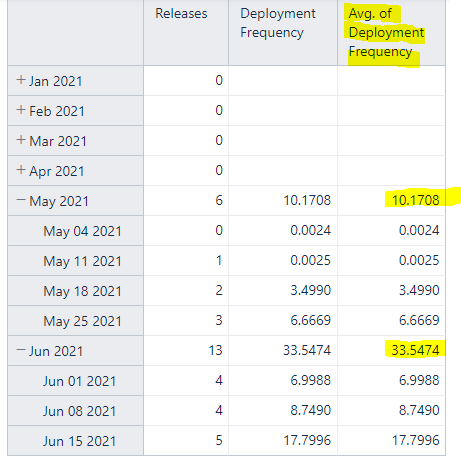# Average is not working for a calculated measure

Hi all,

I’ve been trying many solutions to have an average of the results by month. This is the code that’s not working:
–annotations.group = Deployment Frequency
CASE WHEN [Time].CurrentHierarchyMember.Level.Name = ‘Month’
THEN
Avg(
[Time].CurrentHierarchyMember,
[Measures].[Deployment Frequency]
)

WHEN [Time].CurrentHierarchyMember.Level.Name = ‘Week’
THEN
Avg(
[Time].CurrentHierarchyMember,[Measures].[Deployment Frequency]
)
ELSE
Avg(
[Time].CurrentHierarchyMember,[Measures].[Deployment Frequency]
)
END

Even with the AVG being used the result is a SUM:I’ve tried to make a division by the number of results as a workaround but with no success since I don’t know how to get the number of results being considered for the Time period. Example using Siblings (probably not the solution):
Avg(
[Time].CurrentHierarchyMember,[Measures].[Deployment Frequency]
) / count([Time].CurrentHierarchyMember.Siblings)
ELSE

Many thanks for all, stay healthy, stay safeDanilo

Hi @Danilo ,

The Time dimension current hierarchy member in the formula will consider the Time dimension member currently in rows. Thus it will always be the total sum of the measure value in that period evaluated over that one member.
If you intend to use the “Month” or “Week” level members in rows and want to evaluate the total value for that period over the number of days there were values for the particular measure, the formula could look similar to the one below:

``````Avg(
ChildrenSet([Time].CurrentHierarchyMember),
[Measures].[Issues created]
)
``````

See the picture of the report below:

See more details on the Avg() function on the eazyBI documentation page - Avg - eazyBI.

Best,
Roberts // support@eazybi.com

1 Like

Hi @roberts.cacus many thanks for the explanation. It worked and was exactelly what I needed.
Wish you all the best1 Like